# JEE Main System of Linear Equations Previous Year Questions With Solutions

JEE Previous year set of questions on topic System of Linear Equations gives a clear understanding of the topic. These solutions help in breaking down all the difficult problems by the simple step-by-step method of solving. BYJU’S provides accurate solutions, which are prepared by subject experts. This section deals with the solution of linear systems using different methods. How To Solve a Linear Equation System? Linear equations system can be solved using different methods such as Graphical Method, Elimination Method, Cross Multiplication Method, Substitution Method, Matrix Method and Determinants Method. The set of all possible solutions is called the solution set. A linear system may behave in any one of 3 possible ways: The system has no solution, a single unique solution or infinitely many solutions.

## JEE Main Past Year Questions With Solutions on System of Linear Equations

Question 1: Consider the system of equations x + y + z = 1, 2x + 3y + 2z = 1, 2x + 3y + (a2 – 1)z = a + 1 then

(a) System has a unique solution for |a| = √3

(b) System is inconsistence for |a| = √3

(c) System is inconsistence for a = 4

(d) System is inconsistence for a = 3

Solution:

Given system of linear equations:

x + y + z = 1 ….(1)

2x + 3y + 2z = 1 ….(2)

2x + 3y + (a2 – 1)z = a + 1 …..(3)

Consider a2 – 1 = 2

then LHS of (2) and (3) are same but RHS are not.

Hence a2 = 3 => |a| = √3

For |a| = √3, system is inconsistence.

So option (b) is correct.

Question 2: If the system of linear equations

2x + 2ay + az = 0

2x + 3by + bz = 0 and

2x + 4cy + cz = 0,

where a, b, c Є R are non-zero and distinct; has non-zero solution, then

(a) a + b + c = 0

(b) 1/a, 1/b, 1/c are in A.P.

(c) a, b, c are in A.P.

(d) a, b, c are in G.P.

Solution:

Given system of linear equations

2x + 2ay + az = 0

2x + 3by + bz = 0 and

2x + 4cy + cz = 0,

Now,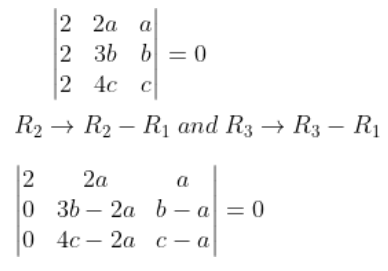=> (3b − 2a)(c − a) − (4c − 2a)(b − a) = 0

=> 3bc − 2ac − 3ab + 2a2 − [4bc − 4ac − 2ab + 2a2] = 0

=> −bc + 2ac − ab = 0

=> ab + bc = 2ac

=> 1/c + 1/a = 2/b

Which shows that 1/a, 1/b, 1/c are in A.P.

Question 3: If system of linear equations

x + y + z = 6

x + 2y + 3z = 10 and

3x + 2y + λz = μ

has more than two solutions, then μ − λ2 is equal to ________.

Solution:

The system of equations has more than 2 solutions.

Find for D = D3 = 0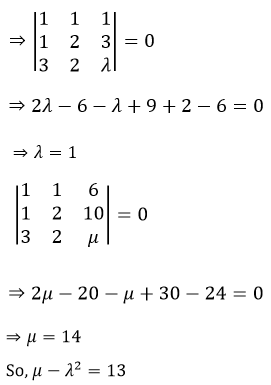Question 4: For which of the following ordered pairs (μ, δ), the system of linear equations

x + 2y + 3z = 1

3x + 4y + 5z = μ

4x + 4y + 4z = δ

is inconsistent?

(a) (4, 6) (b) (3, 4) (c) (1, 0) (d) (4, 3)

Solution: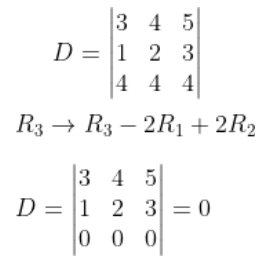For inconsistent system, one of Dx, Dy, Dz should not be equal to 0.

Now,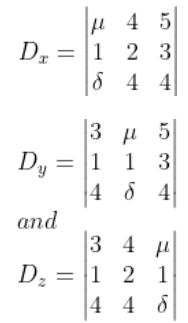For inconsistent system, 2μ ≠ δ + 2

Therefore, the system will be inconsistent for μ = 4, δ = 3.

Question 5: The system of linear equations

λx + 2y + 2z = 5

2λx + 3y + 5z = 8

4x + λy + 6z = 10 has:

(a) no solution when λ = 2

(b) infinitely many solutions when λ = 2

(c) no solution when λ = 8

(d) a unique solution when λ = -8

Solution: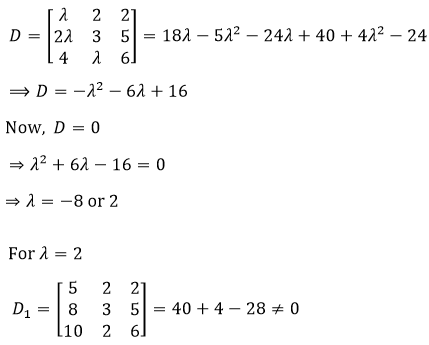Therefore, Equations have no solution for λ= 2.

Question 6: The following system of linear equations

7x + 6y − 2z = 0 ,

3x + 4y + 2z = 0

x − 2y − 6z = 0, has

(a) infinitely many solutions, (x, y, z) satisfying y = 2z

(b) infinitely many solutions, (x, y, z) satisfying x = 2z

(c) no solution

(d) only the trivial solution

Solution:

Given system of linear equations

7x + 6y − 2z = 0 ,

3x + 4y + 2z = 0

x − 2y − 6z = 0,

As the system of equations are Homogeneous

=> The system is consistent.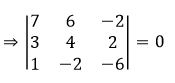=> Infinite solutions exist (both trivial and non-trivial solutions)

When, y = 2z

Let’s take y = 2 and z = 1

When (x, 2, 1)is substituted in the system of equations

=> 7x + 10 = 0,

3x + 10 = 0 and

x − 10 = 0 (which is not possible)

Therefore, y = 2z

=> Infinitely many solutions not exist.

For x = 2z, let’s take x = 2, z = 1, y = y

Substitute (2, y, 1) in system of equations

=> y = −2

So, for each pair of (x, z), we get a value of y.

Therefore, for x = 2z infinitely many solutions exist.

Question 7: If the system of linear equations

x + ky + 3z = 0

3x + ky – 2z = 0 and

2x + 4y – 3z = 0

has a non zero solution (x, y, z) then xz/y2 is equal to

(a) -10 (b) 10 (c) -30 (d) 30

Solution:

Given system of linear equations

x + ky + 3z = 0

3x + ky – 2z = 0 and

2x + 4y – 3z = 0

System has non zero solution, so

D = 0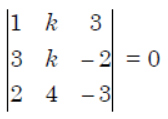1(-3k + 8) – k(-9 + 4) + 3(12 – 2k) = 0

Solving above equation, we have -4k = -44

or k = 11

x + 11y + 3z = 0 …(i)

3x + 11y – 2z = 0 …..(ii)

2x + 4y – 3z = 0 ….(iii)

Solving (i) and (iii)

x = -5y

Using x = -5y in (iii), -10y + 4y – 3z = 0

-6y – 3z = 0

or z = -2y

Now, xz/y2 = (-5y)(-2y)/y2 = 10

Question 8: The number of real values of λ for which the system of linear equations

2x + 4y − λz = 0

4x + λy + 2z = 0

λx + 2y + 2z = 0

has infinitely many solutions, is :

(a) 0 (b) 1 (c) 2 (d) 3

Solution:

For infinitely many solutions, D = 0, Dx = 0, Dy = 0 and Dz = 0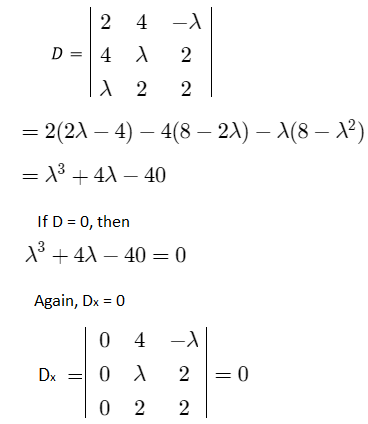Similarly, Dy = 0 and Dz = 0

The equation, λ3 + 4λ – 40 has only one solution.

Since λ(-∞) = -∞ and λ(∞) = ∞

Here α is only one solution, so λ(α) = 0

[Using intermediate value property]

Now, differentiating λ3 + 4λ – 40 w.r.t. λ we get

2 + 4 > 0

The equation can not have y, m, so

λ(m) = 0 and λ(y) = 0

Thus, the number of real values of λ is 1.

Question 9: If x = a, y = b, z = c is a solution of the system of linear equations

x + 8y + 7z = 0

9x + 2y + 3z = 0

x + y + z = 0

such that the point (a, b, c) lies on the plane x + 2y + z = 6, then 2a + b + c equals :

(a) −1 (b) 0 (c) 1 (d) 2

Solution:

Given system of linear equations

x + 8y + 7z = 0 …(i)

9x + 2y + 3z = 0 ….(ii)

x + y + z = 0 ….(iii)

Operate: (ii) – 3 x (iii)

6x – y = 0 or y = 6x …..(iv)

Using (iv) in (i)

x + 8(6x) + 7z = 0

z = -7x ……(v)

Since x = a, y = b, z = c (Given)

b = 6a and c = -7a

Also, (a, b, c) lies on the plane x + 2y + z = 6.

Therefore, a + 2b + c = 6 …..(vi)

Putting the values of b and c in (vi),

a + 2(6a) – 7a = 6

=> a = 1

Also, we get b = 6 and c = -7

Now, 2a + b + c = 2(1) + 6 – 7 = 1

Question 10: It S is the set of distinct values of ‘b’ for which the following system of linear equations x + y + z = 1 x + ay + z = 1 ax + by + z = 0 has no solution, then S is :

(a) an empty set

(b) an infinite set

(c) a finite set containing two or more elements

(d) a singleton

Solution: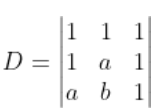= -(a – 1)2 = 0

=> a = 1

We get first two planes co-incident for a = 1.

x + y + z = 1

x + y + z = 1

x + by + z = 0

If b = 1, the system will be inconsistent and hence no solution.

If b ≠ 1, the system will produce infinite solutions.

Hence, for no solution, S has to be a singleton set {1}.